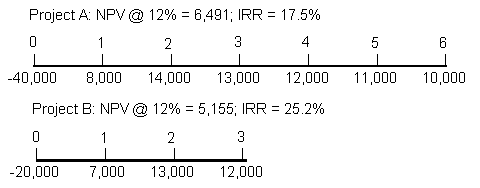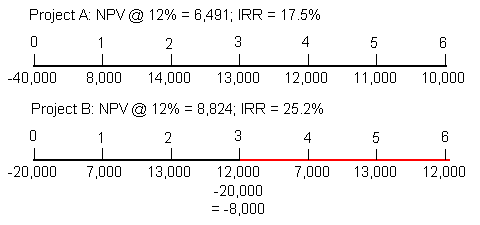### Why should I choose AnalystNotes?

AnalystNotes specializes in helping candidates pass. Period.

##### Subject 3. Project Analysis and Evaluation
If mutually exclusive projects have unequal lives, it may be necessary to adjust the analysis to put the projects on an equal life basis. This can be done using either one of the following approaches. They should lead to the same decisions if consistent assumptions are used.

• Least Common Multiple of Lives Approach: It assumes that each project can be repeated as many times as necessary to reach a common life span; the NPVs over this life span are then compared, and the project with the higher common life NPV is chosen. This approach is easier (than EAA) to explain to decision makers. However, the drawback is that the arithmetic may be too complex.

• Equivalent Annual Annuity (EAA) Approach: It calculates the annual payments a project would provide if it were an annuity. When comparing projects of unequal lives, the one with the higher equivalent annual annuity should be chosen. This approach is easier to apply than the first one.

Note that the unequal life issue does not arise for independent projects.

Suppose XYZ Inc. is planning to modernize its production facilities. It can purchase either a Model A or Model B system. The figure below shows both the expected net cash flows, NPVs and IRRs for these two mutually exclusive projects.The Least Common Multiple of Lives Approach

XYZ Inc. can buy another Model B system in at the end of year 3 to extend the overall combined life of the combined projects to 6 years. We would find the NPV of project B over a six-year period, assuming there's no change in annual cash flows and the cost of capital.The NPV of the extended Model B project over a common life of 6 years is greater than the NPV of the Model A project, so Model B should be chosen.

The Equivalent Annual Annuity Approach

Step 1. Find each project's NPV:

NPVA = \$6,491.
NPVB = \$5,155.

Step 2. For each project, find a constant annuity cash flow (the equivalent annual annuity) that has the same present value of the project's NPV:

For example, EAAB = \$2,146. This level of cash flow stream, when discounted back three years at 12%, has a present value equal to project B's original NPV.

EAAA = \$1,579.

Step 3. The project with a higher EAA should be chosen:

In this example, project B should be chosen.

Capital RationingCapital rationing occurs when management places a constraint on the size of the firm's capital budget during a particular period. In such situations, capital is scarce and should be allocated to the best projects to maximize the firm's aggregate NPV. The firm's capital budget and cost of capital must be determined simultaneously to best allocate the firm's capital.

• In the ideal situation, the budget is adequate to invest in all profitable (positive NPV) projects.
• A common case is that the company has more profitable projects than it can choose. Sometimes the company can choose to invest in the most profitable ones available, but other times it may not be able to due to the size constraint.

Under hard capital rationing, the budget is fixed. In the case of hard rationing, managers use trial and error and sometimes mathematical programming to find the optimal set of projects. In that situation, it is best to use the NPV or PI valuation methods.

Under soft capital rationing, managers may be allowed to over-spend their budgets if they argue effectively that the additional funds will be deployed profitably.

Learning Outcome Statements

c. evaluate capital projects and determine the optimal capital project in situations of 1) mutually exclusive projects with unequal lives, using either the least common multiple of lives approach or the equivalent annual annuity approach, and 2) capital rationing;

CFA® 2023 Level I Curriculum, Volume 3, Module 19

User Comment
kalps This is usually used in replacement analysis therefore the EAA approach should read if the EAA is lower then accept this project
sekib Text on pg 439 of Brigham & Houston

project w/ higher EAA will always have the higher NPV when extended out to any common life therefore chose project w/ higher EAA
sarath Higher EAA is chosen always...first calculate the NPV and then find the corresponding EAA over the time period...
RichardWang How to use BA II to calculate EAA from NPV?
adeelj You can calculate the Annuity Factors using the annuity formula : (1-(1+i)^t)/i
where i is the interest rate(discount rate) and t is the time period.
Hence in the example above we see the annuity factor = (1-(1.12)^(-3))/.12 = 2.40183
Hence EAA equals 5,155 / 2.40183 = 2,146
GIJCFA Using BA II calculator: For Project B,
N=3, I/Y=12, FV=0, PV=NPV=5155, CPT PMT = 2146.28
jmcarr02 PI = NPV/Investment
ShagunSingh Well explained.